Publicité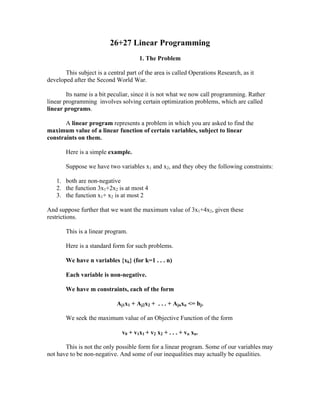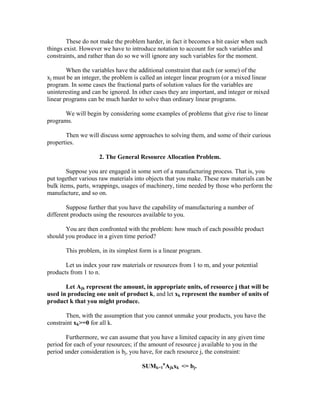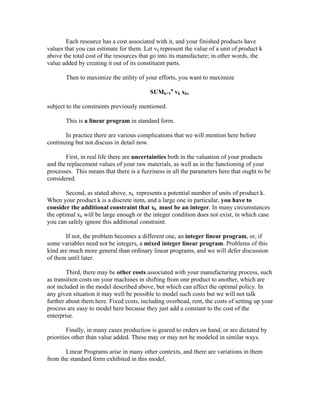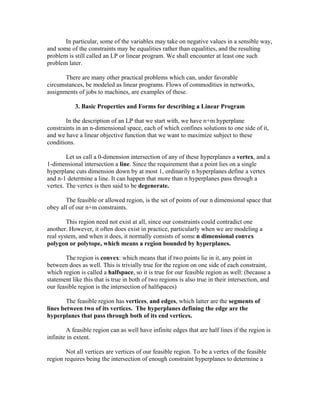Publicité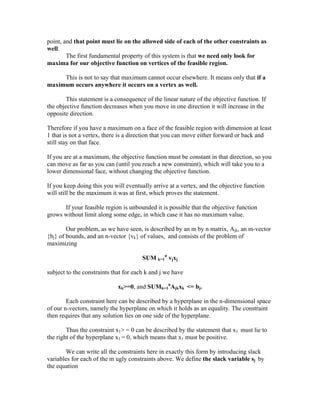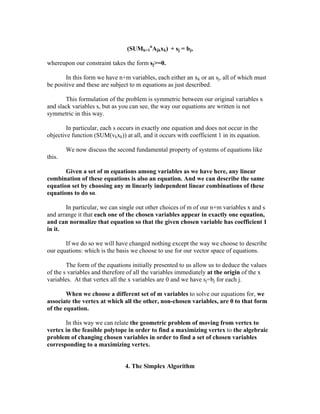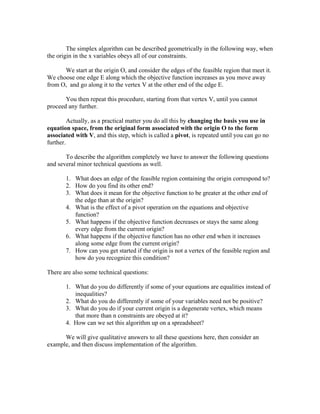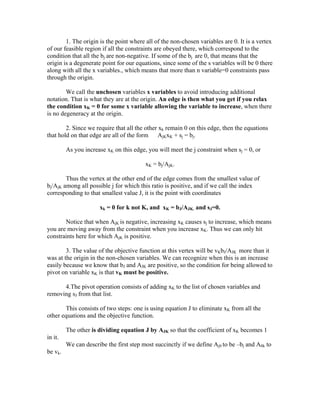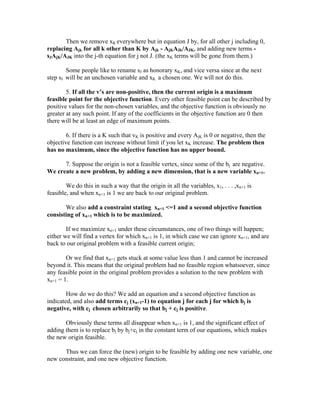Publicité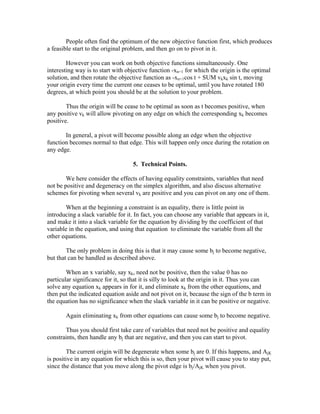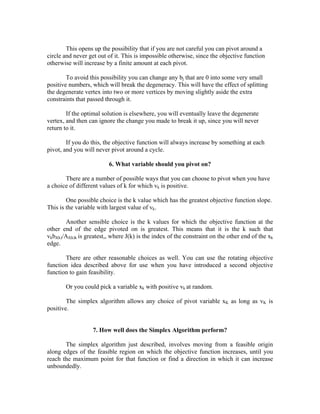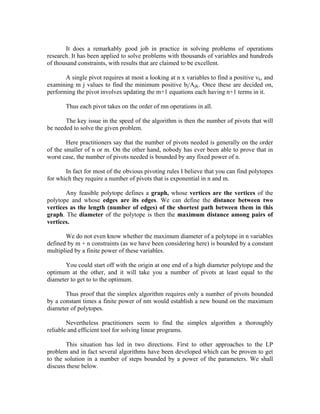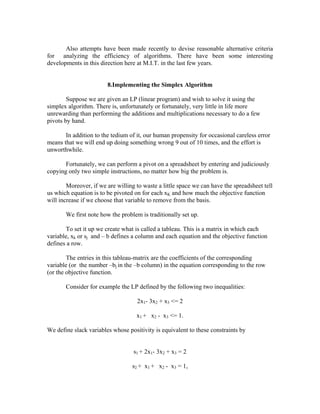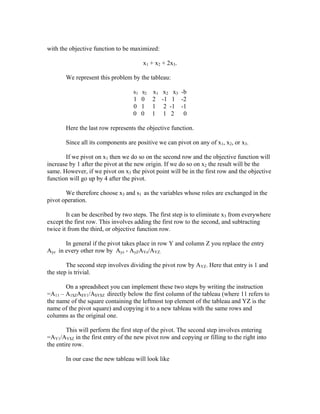Publicité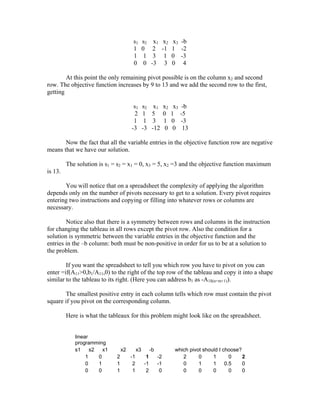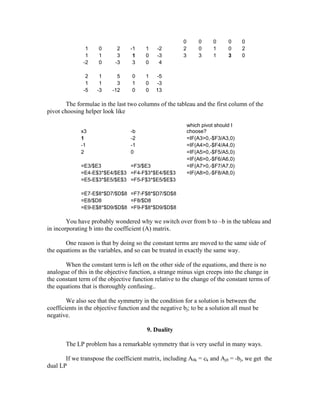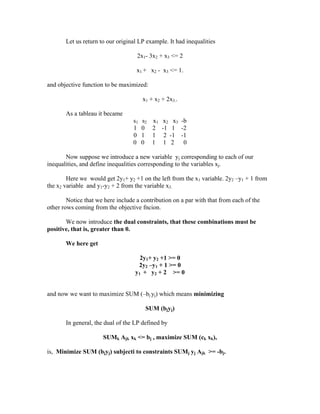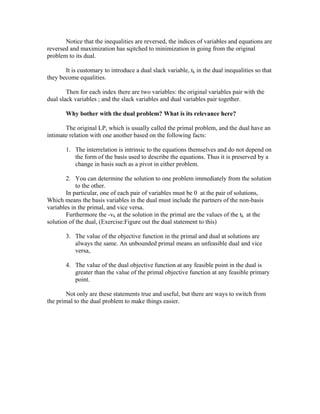Prochain SlideShare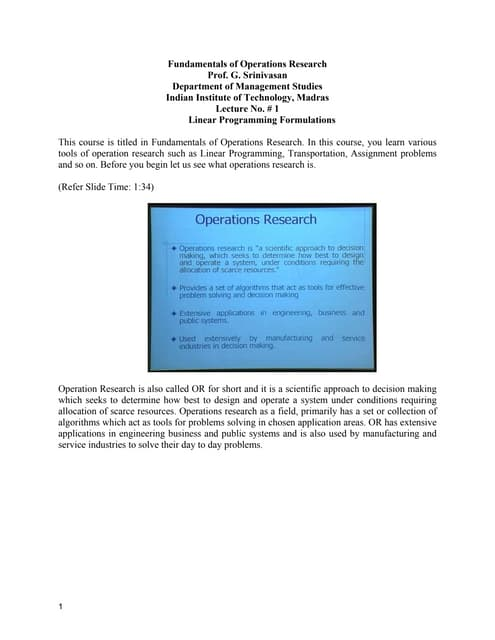Operation Research
Chargement dans ... 3
1 sur 18
Publicité

### Theory of linear programming

1. 26+27 Linear Programming 1. The Problem This subject is a central part of the area is called Operations Research, as it developed after the Second World War. Its name is a bit peculiar, since it is not what we now call programming. Rather linear programming involves solving certain optimization problems, which are called linear programs. A linear program represents a problem in which you are asked to find the maximum value of a linear function of certain variables, subject to linear constraints on them. Here is a simple example. Suppose we have two variables x1 and x2, and they obey the following constraints: 1. both are non-negative 2. the function 3x1+2x2 is at most 4 3. the function x1+ x2 is at most 2 And suppose further that we want the maximum value of 3x1+4x2, given these restrictions. This is a linear program. Here is a standard form for such problems. We have n variables {xk} (for k=1 . . . n) Each variable is non-negative. We have m constraints, each of the form Aj1x1 + Aj2x2 + . . . + Ajnxn <= bj. We seek the maximum value of an Objective Function of the form v0 + v1x1 + v2 x2 + . . . + vn xn. This is not the only possible form for a linear program. Some of our variables may not have to be non-negative. And some of our inequalities may actually be equalities.
2. These do not make the problem harder, in fact it becomes a bit easier when such things exist. However we have to introduce notation to account for such variables and constraints, and rather than do so we will ignore any such variables for the moment. When the variables have the additional constraint that each (or some) of the xj must be an integer, the problem is called an integer linear program (or a mixed linear program. In some cases the fractional parts of solution values for the variables are uninteresting and can be ignored. In other cases they are important, and integer or mixed linear programs can be much harder to solve than ordinary linear programs. We will begin by considering some examples of problems that give rise to linear programs. Then we will discuss some approaches to solving them, and some of their curious properties. 2. The General Resource Allocation Problem. Suppose you are engaged in some sort of a manufacturing process. That is, you put together various raw materials into objects that you make. These raw materials can be bulk items, parts, wrappings, usages of machinery, time needed by those who perform the manufacture, and so on. Suppose further that you have the capability of manufacturing a number of different products using the resources available to you. You are then confronted with the problem: how much of each possible product should you produce in a given time period? This problem, in its simplest form is a linear program. Let us index your raw materials or resources from 1 to m, and your potential products from 1 to n. Let Ajk represent the amount, in appropriate units, of resource j that will be used in producing one unit of product k, and let xk represent the number of units of product k that you might produce. Then, with the assumption that you cannot unmake your products, you have the constraint xk>=0 for all k. Furthermore, we can assume that you have a limited capacity in any given time period for each of your resources; if the amount of resource j available to you in the period under consideration is bj, you have, for each resource j, the constraint: SUMk=1nAjkxk <= bj.
4. In particular, some of the variables may take on negative values in a sensible way, and some of the constraints may be equalities rather than equalities, and the resulting problem is still called an LP or linear program. We shall encounter at least one such problem later. There are many other practical problems which can, under favorable circumstances, be modeled as linear programs. Flows of commodities in networks, assignments of jobs to machines, are examples of these. 3. Basic Properties and Forms for describing a Linear Program In the description of an LP that we start with, we have n+m hyperplane constraints in an n-dimensional space, each of which confines solutions to one side of it, and we have a linear objective function that we want to maximize subject to these conditions. Let us call a 0-dimension intersection of any of these hyperplanes a vertex, and a 1-dimensional intersection a line. Since the requirement that a point lies on a single hyperplane cuts dimension down by at most 1, ordinarily n hyperplanes define a vertex and n-1 determine a line. It can happen that more than n hyperplanes pass through a vertex. The vertex is then said to be degenerate. The feasible or allowed region, is the set of points of our n dimensional space that obey all of our n+m constraints. This region need not exist at all, since our constraints could contradict one another. However, it often does exist in practice, particularly when we are modeling a real system, and when it does, it normally consists of some n dimensional convex polygon or polytope, which means a region bounded by hyperplanes. The region is convex: which means that if two points lie in it, any point in between does as well. This is trivially true for the region on one side of each constraint, which region is called a halfspace, so it is true for our feasible region as well: (because a statement like this that is true in both of two regions is also true in their intersection, and our feasible region is the intersection of halfspaces) The feasible region has vertices, and edges, which latter are the segments of lines between two of its vertices. The hyperplanes defining the edge are the hyperplanes that pass through both of its end vertices. A feasible region can as well have infinite edges that are half lines if the region is infinite in extent. Not all vertices are vertices of our feasible region. To be a vertex of the feasible region requires being the intersection of enough constraint hyperplanes to determine a
5. point, and that point must lie on the allowed side of each of the other constraints as well. The first fundamental property of this system is that we need only look for maxima for our objective function on vertices of the feasible region. This is not to say that maximum cannot occur elsewhere. It means only that if a maximum occurs anywhere it occurs on a vertex as well. This statement is a consequence of the linear nature of the objective function. If the objective function decreases when you move in one direction it will increase in the opposite direction. Therefore if you have a maximum on a face of the feasible region with dimension at least 1 that is not a vertex, there is a direction that you can move either forward or back and still stay on that face. If you are at a maximum, the objective function must be constant in that direction, so you can move as far as you can (until you reach a new constraint), which will take you to a lower dimensional face, without changing the objective function. If you keep doing this you will eventually arrive at a vertex, and the objective function will still be the maximum it was at first, which proves the statement. If your feasible region is unbounded it is possible that the objective function grows without limit along some edge, in which case it has no maximum value. Our problem, as we have seen, is described by an m by n matrix, Ajk, an m-vector {bj} of bounds, and an n-vector {vk} of values, and consists of the problem of maximizing SUM k=1n vjxj subject to the constraints that for each k and j we have xk>=0, and SUMk=1nAjkxk <= bj. Each constraint here can be described by a hyperplane in the n-dimensional space of our n-vectors, namely the hyperplane on which it holds as an equality. The constraint then requires that any solution lies on one side of the hyperplane. Thus the constraint x1> = 0 can be described by the statement that x1 must lie to the right of the hyperplane x1 = 0, which means that x1 must be positive. We can write all the constraints here in exactly this form by introducing slack variables for each of the m ugly constraints above. We define the slack variable sj by the equation
6. (SUMk=1nAjkxk) + sj = bj, whereupon our constraint takes the form sj>=0. In this form we have n+m variables, each either an xk or an sj, all of which must be positive and these are subject to m equations as just described. This formulation of the problem is symmetric between our original variables x and slack variables s, but as you can see, the way our equations are written is not symmetric in this way. In particular, each s occurs in exactly one equation and does not occur in the objective function (SUM(vkxk)) at all, and it occurs with coefficient 1 in its equation. We now discuss the second fundamental property of systems of equations like this. Given a set of m equations among variables as we have here, any linear combination of these equations is also an equation. And we can describe the same equation set by choosing any m linearly independent linear combinations of these equations to do so. In particular, we can single out other choices of m of our n+m variables x and s and arrange it that each one of the chosen variables appear in exactly one equation, and can normalize that equation so that the given chosen variable has coefficient 1 in it. If we do so we will have changed nothing except the way we choose to describe our equations: which is the basis we choose to use for our vector space of equations. The form of the equations initially presented to us allow us to deduce the values of the s variables and therefore of all the variables immediately at the origin of the x variables. At that vertex all the x variables are 0 and we have sj=bj for each j. When we choose a different set of m variables to solve our equations for, we associate the vertex at which all the other, non-chosen variables, are 0 to that form of the equation. In this way we can relate the geometric problem of moving from vertex to vertex in the feasible polytope in order to find a maximizing vertex to the algebraic problem of changing chosen variables in order to find a set of chosen variables corresponding to a maximizing vertex. 4. The Simplex Algorithm
7. The simplex algorithm can be described geometrically in the following way, when the origin in the x variables obeys all of our constraints. We start at the origin O, and consider the edges of the feasible region that meet it. We choose one edge E along which the objective function increases as you move away from O, and go along it to the vertex V at the other end of the edge E. You then repeat this procedure, starting from that vertex V, until you cannot proceed any further. Actually, as a practical matter you do all this by changing the basis you use in equation space, from the original form associated with the origin O to the form associated with V, and this step, which is called a pivot, is repeated until you can go no further. To describe the algorithm completely we have to answer the following questions and several minor technical questions as well. 1. What does an edge of the feasible region containing the origin correspond to? 2. How do you find its other end? 3. What does it mean for the objective function to be greater at the other end of the edge than at the origin? 4. What is the effect of a pivot operation on the equations and objective function? 5. What happens if the objective function decreases or stays the same along every edge from the current origin? 6. What happens if the objective function has no other end when it increases along some edge from the current origin? 7. How can you get started if the origin is not a vertex of the feasible region and how do you recognize this condition? There are also some technical questions: 1. What do you do differently if some of your equations are equalities instead of inequalities? 2. What do you do differently if some of your variables need not be positive? 3. What do you do if your current origin is a degenerate vertex, which means that more than n constraints are obeyed at it? 4. How can we set this algorithm up on a spreadsheet? We will give qualitative answers to all these questions here, then consider an example, and then discuss implementation of the algorithm.
8. 1. The origin is the point where all of the non-chosen variables are 0. It is a vertex of our feasible region if all the constraints are obeyed there, which correspond to the condition that all the bj are non-negative. If some of the bj are 0, that means that the origin is a degenerate point for our equations, since some of the s variables will be 0 there along with all the x variables., which means that more than n variable=0 constraints pass through the origin. We call the unchosen variables x variables to avoid introducing additional notation. That is what they are at the origin. An edge is then what you get if you relax the condition xK = 0 for some x variable allowing the variable to increase, when there is no degeneracy at the origin. 2. Since we require that all the other xk remain 0 on this edge, then the equations that hold on that edge are all of the form AjKxK + sj = bj. As you increase xK on this edge, you will meet the j constraint when sj = 0, or xK = bj/AjK. Thus the vertex at the other end of the edge comes from the smallest value of bj/AjK among all possible j for which this ratio is positive, and if we call the index corresponding to that smallest value J, it is the point with coordinates xk = 0 for k not K, and xK = bJ/AJK. and sJ=0. Notice that when AjK is negative, increasing xK causes sj to increase, which means you are moving away from the constraint when you increase xK. Thus we can only hit constraints here for which AjK is positive. 3. The value of the objective function at this vertex will be vKbJ/AJK more than it was at the origin in the non-chosen variables. We can recognize when this is an increase easily because we know that bJ and AJK are positive, so the condition for being allowed to pivot on variable xK is that vK must be positive. 4.The pivot operation consists of adding xK to the list of chosen variables and removing sJ from that list. This consists of two steps: one is using equation J to eliminate xK from all the other equations and the objective function. The other is dividing equation J by AJK so that the coefficient of xK becomes 1 in it. We can describe the first step most succinctly if we define Aj0 to be –bj and A0k to be vk.
9. Then we remove xK everywhere but in equation J by, for all other j including 0, replacing Ajk for all k other than K by Ajk - AjKAJk/AJK, and adding new terms - sJAjK/AJK into the j-th equation for j not J. (the xK terms will be gone from them.) Some people like to rename sJ as honorary xK, and vice versa since at the next step sJ will be an unchosen variable and xK a chosen one. We will not do this. 5. If all the v’s are non-positive, then the current origin is a maximum feasible point for the objective function. Every other feasible point can be described by positive values for the non-chosen variables, and the objective function is obviously no greater at any such point. If any of the coefficients in the objective function are 0 then there will be at least an edge of maximum points. 6. If there is a K such that vK is positive and every AjK is 0 or negative, then the objective function can increase without limit if you let xK increase. The problem then has no maximum, since the objective function has no upper bound. 7. Suppose the origin is not a feasible vertex, since some of the bj are negative. We create a new problem, by adding a new dimension, that is a new variable xn+1. We do this in such a way that the origin in all the variables, x1, . . . ,xn+1 is feasible, and when xn+1 is 1 we are back to our original problem. We also add a constraint stating xn+1 <=1 and a second objective function consisting of xn+1 which is to be maximized. If we maximize xn+1 under these circumstances, one of two things will happen; either we will find a vertex for which xn+1 is 1, in which case we can ignore xn+1, and are back to our original problem with a feasible current origin; Or we find that xn+1 gets stuck at some value less than 1 and cannot be increased beyond it. This means that the original problem had no feasible region whatsoever, since any feasible point in the original problem provides a solution to the new problem with xn+1 = 1. How do we do this? We add an equation and a second objective function as indicated, and also add terms cj (xn+1-1) to equation j for each j for which bj is negative, with cj chosen arbitrarily so that bj + cj is positive. Obviously these terms all disappear when xn+1 is 1, and the significant effect of adding them is to replace bj by bj+cj in the constant term of our equations, which makes the new origin feasible. Thus we can force the (new) origin to be feasible by adding one new variable, one new constraint, and one new objective function.
10. People often find the optimum of the new objective function first, which produces a feasible start to the original problem, and then go on to pivot in it. However you can work on both objective functions simultaneously. One interesting way is to start with objective function -xn+1 for which the origin is the optimal solution, and then rotate the objective function as -xn+1cos t + SUM vkxk sin t, moving your origin every time the current one ceases to be optimal, until you have rotated 180 degrees, at which point you should be at the solution to your problem. Thus the origin will be cease to be optimal as soon as t becomes positive, when any positive vk will allow pivoting on any edge on which the corresponding xk becomes positive. In general, a pivot will become possible along an edge when the objective function becomes normal to that edge. This will happen only once during the rotation on any edge. 5. Technical Points. We here consider the effects of having equality constraints, variables that need not be positive and degeneracy on the simplex algorithm, and also discuss alternative schemes for pivoting when several vk are positive and you can pivot on any one of them. When at the beginning a constraint is an equality, there is little point in introducing a slack variable for it. In fact, you can choose any variable that appears in it, and make it into a slack variable for the equation by dividing by the coefficient of that variable in the equation, and using that equation to eliminate the variable from all the other equations. The only problem in doing this is that it may cause some bj to become negative, but that can be handled as described above. When an x variable, say xk, need not be positive, then the value 0 has no particular significance for it, so that it is silly to look at the origin in it. Thus you can solve any equation xk appears in for it, and eliminate xk from the other equations, and then put the indicated equation aside and not pivot on it, because the sign of the b term in the equation has no significance when the slack variable in it can be positive or negative. Again eliminating xk from other equations can cause some bj to become negative. Thus you should first take care of variables that need not be positive and equality constraints, then handle any bj that are negative, and then you can start to pivot. The current origin will be degenerate when some bj are 0. If this happens, and AjK is positive in any equation for which this is so, then your pivot will cause you to stay put, since the distance that you move along the pivot edge is bj/AjK when you pivot.
11. This opens up the possibility that if you are not careful you can pivot around a circle and never get out of it. This is impossible otherwise, since the objective function otherwise will increase by a finite amount at each pivot. To avoid this possibility you can change any bj that are 0 into some very small positive numbers, which will break the degeneracy. This will have the effect of splitting the degenerate vertex into two or more vertices by moving slightly aside the extra constraints that passed through it. If the optimal solution is elsewhere, you will eventually leave the degenerate vertex, and then can ignore the change you made to break it up, since you will never return to it. If you do this, the objective function will always increase by something at each pivot, and you will never pivot around a cycle. 6. What variable should you pivot on? There are a number of possible ways that you can choose to pivot when you have a choice of different values of k for which vk is positive. One possible choice is the k value which has the greatest objective function slope. This is the variable with largest value of vk. Another sensible choice is the k values for which the objective function at the other end of the edge pivoted on is greatest. This means that it is the k such that vkbJ(k)/AJ(k)k is greatest,, where J(k) is the index of the constraint on the other end of the xk edge. There are other reasonable choices as well. You can use the rotating objective function idea described above for use when you have introduced a second objective function to gain feasibility. Or you could pick a variable xk with positive vk at random. The simplex algorithm allows any choice of pivot variable xK as long as vK is positive. 7. How well does the Simplex Algorithm perform? The simplex algorithm just described, involves moving from a feasible origin along edges of the feasible region on which the objective function increases, until you reach the maximum point for that function or find a direction in which it can increase unboundedly.
12. It does a remarkably good job in practice in solving problems of operations research. It has been applied to solve problems with thousands of variables and hundreds of thousand constraints, with results that are claimed to be excellent. A single pivot requires at most a looking at n x variables to find a positive vk, and examining m j values to find the minimum positive bj/AjK. Once these are decided on, performing the pivot involves updating the m+1 equations each having n+1 terms in it. Thus each pivot takes on the order of mn operations in all. The key issue in the speed of the algorithm is then the number of pivots that will be needed to solve the given problem. Here practitioners say that the number of pivots needed is generally on the order of the smaller of n or m. On the other hand, nobody has ever been able to prove that in worst case, the number of pivots needed is bounded by any fixed power of n. In fact for most of the obvious pivoting rules I believe that you can find polytopes for which they require a number of pivots that is exponential in n and m. Any feasible polytope defines a graph, whose vertices are the vertices of the polytope and whose edges are its edges. We can define the distance between two vertices as the length (number of edges) of the shortest path between them in this graph. The diameter of the polytope is then the maximum distance among pairs of vertices. We do not even know whether the maximum diameter of a polytope in n variables defined by m + n constraints (as we have been considering here) is bounded by a constant multiplied by a finite power of these variables. You could start off with the origin at one end of a high diameter polytope and the optimum at the other, and it will take you a number of pivots at least equal to the diameter to get to to the optimum. Thus proof that the simplex algorithm requires only a number of pivots bounded by a constant times a finite power of nm would establish a new bound on the maximum diameter of polytopes. Nevertheless practitioners seem to find the simplex algorithm a thoroughly reliable and efficient tool for solving linear programs. This situation has led in two directions. First to other approaches to the LP problem and in fact several algorithms have been developed which can be proven to get to the solution in a number of steps bounded by a power of the parameters. We shall discuss these below.
13. Also attempts have been made recently to devise reasonable alternative criteria for analyzing the efficiency of algorithms. There have been some interesting developments in this direction here at M.I.T. in the last few years. 8.Implementing the Simplex Algorithm Suppose we are given an LP (linear program) and wish to solve it using the simplex algorithm. There is, unfortunately or fortunately, very little in life more unrewarding than performing the additions and multiplications necessary to do a few pivots by hand. In addition to the tedium of it, our human propensity for occasional careless error means that we will end up doing something wrong 9 out of 10 times, and the effort is unworthwhile. Fortunately, we can perform a pivot on a spreadsheet by entering and judiciously copying only two simple instructions, no matter how big the problem is. Moreover, if we are willing to waste a little space we can have the spreadsheet tell us which equation is to be pivoted on for each xK and how much the objective function will increase if we choose that variable to remove from the basis. We first note how the problem is traditionally set up. To set it up we create what is called a tableau. This is a matrix in which each variable, xk or sj and – b defines a column and each equation and the objective function defines a row. The entries in this tableau-matrix are the coefficients of the corresponding variable (or the number –bj in the –b column) in the equation corresponding to the row (or the objective function. Consider for example the LP defined by the following two inequalities: 2x1- 3x2 + x3 <= 2 x1 + x2 - x3 <= 1. We define slack variables whose positivity is equivalent to these constraints by s1 + 2x1- 3x2 + x3 = 2 s2 + x1 + x2 - x3 = 1,
14. with the objective function to be maximized: x1 + x2 + 2x3. We represent this problem by the tableau: s1 s2 x1 x2 x3 -b 1 0 2 -1 1 -2 0 1 1 2 -1 -1 0 0 1 1 2 0 Here the last row represents the objective function. Since all its components are positive we can pivot on any of x1, x2, or x3. If we pivot on x1 then we do so on the second row and the objective function will increase by 1 after the pivot at the new origin. If we do so on x2 the result will be the same. However, if we pivot on x3 the pivot point will be in the first row and the objective function will go up by 4 after the pivot. We therefore choose x3 and s1 as the variables whose roles are exchanged in the pivot operation. It can be described by two steps. The first step is to eliminate x3 from everywhere except the first row. This involves adding the first row to the second, and subtracting twice it from the third, or objective function row. In general if the pivot takes place in row Y and column Z you replace the entry Ayz in every other row by Ayz - AyZAYz/AYZ. The second step involves dividing the pivot row by AYZ. Here that entry is 1 and the step is trivial. On a spreadsheet you can implement these two steps by writing the instruction =A11 – A1\$ZA\$Y1/A\$Y\$Z directly below the first column of the tableau (where 11 refers to the name of the square containing the leftmost top element of the tableau and YZ is the name of the pivot square) and copying it to a new tableau with the same rows and columns as the original one. This will perform the first step of the pivot. The second step involves entering =AY1/AY\$Z in the first entry of the new pivot row and copying or filling to the right into the entire row. In our case the new tableau will look like
15. s1 s2 x1 x2 x3 -b 1 0 2 -1 1 -2 1 1 3 1 0 -3 0 0 -3 3 0 4 At this point the only remaining pivot possible is on the column x2 and second row. The objective function increases by 9 to 13 and we add the second row to the first, getting s1 s2 x1 x2 x3 -b 2 1 5 0 1 -5 1 1 3 1 0 -3 -3 -3 -12 0 0 13 Now the fact that all the variable entries in the objective function row are negative means that we have our solution. The solution is s1 = s2 = x1 = 0, x3 = 5, x2 =3 and the objective function maximum is 13. You will notice that on a spreadsheet the complexity of applying the algorithm depends only on the number of pivots necessary to get to a solution. Every pivot requires entering two instructions and copying or filling into whatever rows or columns are necessary. Notice also that there is a symmetry between rows and columns in the instruction for changing the tableau in all rows except the pivot row. Also the condition for a solution is symmetric between the variable entries in the objective function and the entries in the –b column: both must be non-positive in order for us to be at a solution to the problem. If you want the spreadsheet to tell you which row you have to pivot on you can enter =if(A11>0,b1/A11,0) to the right of the top row of the tableau and copy it into a shape similar to the tableau to its right. (Here you can address b1 as -A1\$(n+m+1)). The smallest positive entry in each column tells which row must contain the pivot square if you pivot on the corresponding column. Here is what the tableaux for this problem might look like on the spreadsheet. linear programming s1 s2 x1 x2 x3 -b which pivot should I choose? 1 0 2 -1 1 -2 2 0 1 0 2 0 1 1 2 -1 -1 0 1 1 0.5 0 0 0 1 1 2 0 0 0 0 0 0
16. 0 0 0 0 0 1 0 2 -1 1 -2 2 0 1 0 2 1 1 3 1 0 -3 3 3 1 3 0 -2 0 -3 3 0 4 2 1 5 0 1 -5 1 1 3 1 0 -3 -5 -3 -12 0 0 13 The formulae in the last two columns of the tableau and the first column of the pivot choosing helper look like which pivot should I x3 -b choose? 1 -2 =IF(A3>0,-\$F3/A3,0) -1 -1 =IF(A4>0,-\$F4/A4,0) 2 0 =IF(A5>0,-\$F5/A5,0) =IF(A6>0,-\$F6/A6,0) =E3/\$E3 =F3/\$E3 =IF(A7>0,-\$F7/A7,0) =E4-E\$3*\$E4/\$E\$3 =F4-F\$3*\$E4/\$E\$3 =IF(A8>0,-\$F8/A8,0) =E5-E\$3*\$E5/\$E\$3 =F5-F\$3*\$E5/\$E\$3 =E7-E\$8*\$D7/\$D\$8 =F7-F\$8*\$D7/\$D\$8 =E8/\$D8 =F8/\$D8 =E9-E\$8*\$D9/\$D\$8 =F9-F\$8*\$D9/\$D\$8 You have probably wondered why we switch over from b to –b in the tableau and in incorporating b into the coefficient (A) matrix. One reason is that by doing so the constant terms are moved to the same side of the equations as the variables, and so can be treated in exactly the same way. When the constant term is left on the other side of the equations, and there is no analogue of this in the objective function, a strange minus sign creeps into the change in the constant term of the objective function relative to the change of the constant terms of the equations that is thoroughly confusing.. We also see that the symmetry in the condition for a solution is between the coefficients in the objective function and the negative bj; to be a solution all must be negative. 9. Duality The LP problem has a remarkable symmetry that is very useful in many ways. If we transpose the coefficient matrix, including A0k = ck and Aj0 = -bj, we get the dual LP
17. Let us return to our original LP example. It had inequalities 2x1- 3x2 + x3 <= 2 x1 + x2 - x3 <= 1. and objective function to be maximized: x1 + x2 + 2x3.. As a tableau it became s1 s2 x1 x2 x3 -b 1 0 2 -1 1 -2 0 1 1 2 -1 -1 0 0 1 1 2 0 Now suppose we introduce a new variable yj corresponding to each of our inequalities, and define inequalities corresponding to the variables xj. Here we would get 2y1+ y2 +1 on the left from the x1 variable. 2y2 –y1 + 1 from the x2 variable and y1-y2 + 2 from the variable x3. Notice that we here include a contribution on a par with that from each of the other rows coming from the objective fncion. We now introduce the dual constraints, that these combinations must be positive, that is, greater than 0. We here get 2y1+ y2 +1 >= 0 2y2 –y1 + 1 >= 0 y1 + y2 + 2 >= 0 and now we want to maximize SUM (–bj yj) which means minimizing SUM (bjyj) In general, the dual of the LP defined by SUMk Ajk xk <= bj , maximize SUM (ck xk), is, Minimize SUM (bjyj) subjecti to constraints SUMj yj Ajk >= -bj.
18. Notice that the inequalities are reversed, the indices of variables and equations are reversed and maximization has sqitched to minimization in going from the original problem to its dual. It is customary to introduce a dual slack variable, tk in the dual inequalities so that they become equalities. Then for each index there are two variables: the original variables pair with the dual slack variables ; and the slack variables and dual variables pair together. Why bother with the dual problem? What is its relevance here? The original LP, which is usually called the primal problem, and the dual have an intimate relation with one another based on the following facts: 1. The interrelation is intrinsic to the equations themselves and do not depend on the form of the basis used to describe the equations. Thus it is preserved by a change in basis such as a pivot in either problem. 2. You can determine the solution to one problem immediately from the solution to the other. In particular, one of each pair of variables must be 0 at the pair of solutions, Which means the basis variables in the dual must include the partners of the non-basis variables in the primal, and vice versa. Furthermore the -vk at the solution in the primal are the values of the tk at the solution of the dual, (Exercise:Figure out the dual statement to this) 3. The value of the objective function in the primal and dual at solutions are always the same. An unbounded primal means an unfeasible dual and vice versa, 4. The value of the dual objective function at any feasible point in the dual is greater than the value of the primal objective function at any feasible primary point. Not only are these statements true and useful, but there are ways to switch from the primal to the dual problem to make things easier.
Publicité